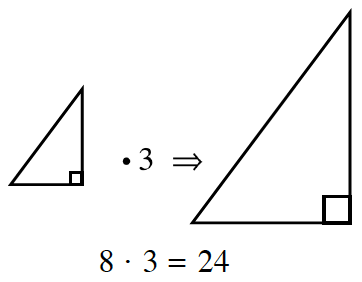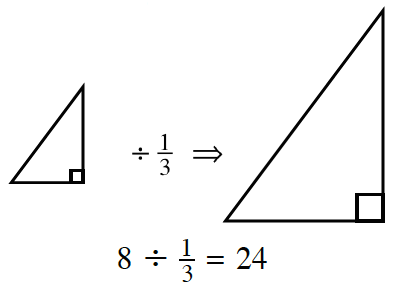Home > CCAA > Chapter cc36 > Lesson cc36.2.5 > Problem6-93

6-93.

Alex and Maria were trying to find the side labeled $x$ in problem 6-92. Their work is shown below. Homework Help ✎

 Alex: “I noticed that when I multiplied by $3$, the sides of the triangle got longer.”Maria: “I remember that when we were dilating shapes in Lesson 6.2.2, my shape got bigger when I divided by $\frac { 1 } { 3 }$.”1. Look at each student’s work. Why do both multiplying by $3$ and dividing by $\frac { 1 } { 3 }$ make the triangles larger?

Since we know that multiplication and division are opposite operations, how can we explain why this happens?

Consider what happens when you multiply a shape by a number less than $1$; the shape becomes smaller.
On the other hand, dividing by a number larger than $1$ will also cause a shape to become smaller.
Are the two expressions above any different?

2. Use Alex and Maria’s strategy to write two expressions to find the value of $y$ in problem 6-92.

Try using the same factors as Alex and Maria to multiply or divide by the appropriate side length to find the value of $y$. Refer to Problem 6-94 for help.

Use the side length $30$ as the multiple or divisor.

$30\div3=10$

$30\left(\frac{1}{3}\right)=10$